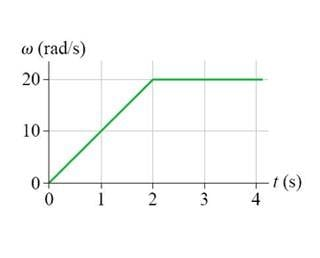# Angular Velocity and Acceleration graph

## Homework StatementA.) For the graph above what is the angular displacement during the 4 seconds of motion?
B.) For the graph above what is the angular acceleration from t=2 to t=4?

## Homework Equations

α = at / r
α = ω / t
α = Θ / t^2
ω = Θ / t
ω = v / r
Θ = ω t + 0.5 α t^2

## The Attempt at a Solution

A.) I used ω = Θ / t
rearranged it to ωt = Θ

but my issue is what ω do i use? 0, 10, 20? anything in between? And is this even the right equation to use?

B.) Isn't it not accelerating between t2 and t4? Or is it constant acceleration?
How i went about it is i used α = ω / t
since the time is 2 seconds, and the ω is 20. i got 10, but that was wrong :(

ProfuselyQuarky
Gold Member
There is an error in your image, unfortunately.

There is an error in your image, unfortunately.
fixed it

For the first part it is the area under the graph.
For the second part the angular acceleration is constant.

haruspex
Homework Helper
Gold Member
what ω do i use?
Do you know how to find the distance moved from a velocity-time graph?
α = ω / t
Quoting formulae is of little use unless you know what the terms in the formulae represent. E.g. a=v/t is more informatively written as aavg=Δv/Δt. I.e. the average acceleration is the increase in velocity divided by the elapsed time.
For the second part the angular acceleration is constant
True, but of the choice
not accelerating between t2 and t4? Or is it constant acceleration?